The calculators on this page require JavaScript but you appear to have switched JavaScript off (it is disabled). Please go to the Preferences for this browser and enable it if you want to use the calculators, then Reload this page.

# The Lucas Numbers

We have seen in earlier pages that there is another series quite similar to the Fibonacci series that often occurs when working with the Fibonacci series. Edouard Lucas (1842-1891) (who gave the name "Fibonacci Numbers" to the series written about by Leonardo of Pisa) studied this second series of numbers: 2, 1, 3, 4, 7, 11, 18, .. called the Lucas numbers in his honour. On this page we examine some of the interesting properties of the Lucas numbers themselves as well as looking at its close relationship with the Fibonacci numbers. The following page generalises further by taking any two starting values.

# Contents

The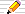icon means there are You do the maths... investigations at the end of that section.
The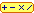calculator icon indicates an interactive calculator in that section.

2, 1, 3, 4, 7, 11, 18, 29, 47, 76, 123, 199, 322, 521, 843 ..More..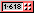## Other starting values for a "Fibonacci" series

The definition of the Fibonacci series is:
Fn+1 = Fn-1 + Fn , if n>1
F0 = 0
F1 = 1
What if we have the same general rule: add the latest two values to get the next but we started with different values instead of 0 and 1?

###You do the maths...

1. The Fibonacci series starts with 0 and 1. What if we started a "Fibonacci" series with 1 and 2, using the same general rule is for the Fibonacci series proper, so that F0 = 1 and F1 = 2? What numbers follow?
The Fibonacci numbers!
2. What if we started with 2 and 3 so that F0 = 2 and F1 = 3?
3. What other starting values give the same series as the previous two questions?
0 and 1, or
1 and 1, or
1 and 2 or even
1 and 0 (in this order)
all of which we recognise as (part of) the Fibonacci series after a few terms.
The next two simplest numbers are 2 and 1.
What if we started with 2 and 1 so that F0 = 2 and F1 = 1? Does this become part of the FIbonacci series too?
5. Try some other starting values of your own.
Here is a calculator to find these terms
6. Investigate what happens to the ratio of successive terms in the series of the earlier questions. We know that for the Fibonacci series, the ratio gets closer and closer to Phi = (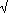5+1)/2. Does it look as (oh dear, I feel a pun coming on: Lucas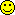) if all the series, no matter what starting values we choose, eventually have successive terms whose ratio is Phi?
Yes! No matter what values we start with, positive or negative, the ratio of two neighbouring terms will either be Phi or else -phi

2, 1, 3, 4, 7, 11, 18, 29, 47, 76, 123, 199, 322, 521, 843 ..More..## The Lucas series

The French mathematician, Edouard Lucas (1842-1891), who gave the series of numbers 0, 1, 1, 2, 3, 5, 8, 13, .. the name the Fibonacci Numbers, found a similar series occurs often when he was investigating Fibonacci number patterns:
2, 1, 3, 4, 7, 11, 18, ...
The Fibonacci rule of adding the latest two to get the next is kept, but here we start from 2 and 1 (in this order) instead of 0 and 1 for the (ordinary) Fibonacci numbers.
The series, called the Lucas Numbers after him, is defined as follows: where we write its members as Ln, for Lucas:
Ln = Ln-1 + Ln-2 for n>1
L0 = 2
L1 = 1
and here are some more values of Ln together with the Fibonacci numbers for comparison:
n: 0 1 2 3 4 5 6 7 8 9 10 ...
Fn: 0 1 1 2 3 5 8 13 21 34 55 ...
Ln: 2 1 3 4 7 11 18 29 47 76 123 ...
The Lucas numbers have lots of properties similar to those of Fibonacci numbers and, uniquely among the series you examined in the You do the maths... section above, the Lucas numbers often occur in various formulae for the Fibonacci Numbers. Also, if you look at many formulae for the Lucas numbers, you will find the Fibonacci series is there too. The next section introduces you to some of these equations. So of all the 'general Fibonacci' series, these two seem to be the most important.

For instance, here is the graph of the ratios of successive Lucas numbers:

 1 = 0·52
 3 = 31
 4 = 1·333..3
 7 = 1·754
 11 = 1·5714..7
 18 = 1·6363..11
 29 = 1·6111..18
 47 = 1·6206..29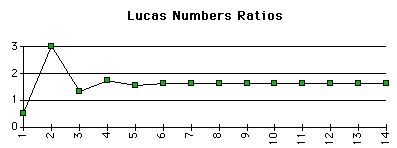In fact, for every series formed by adding the latest two values to get the next, and no matter what two positive values we start with we will always end up having terms whose ratio is Phi=1·6180339.. eventually!

2, 1, 3, 4, 7, 11, 18, 29, 47, 76, 123, 199, 322, 521, 843 ..More..## Two formulae relating the Lucas and Fibonacci numbers

Suppose we add up alternate Fibonacci numbers, Fn-1 + Fn+1; that is, what do you notice about the two Fibonacci numbers either side of a Lucas number in the table below? Tap (click) on a grayed Lucas number to highlight the neighbouring Fibonacci numbers.
n: 0 1 2 3 4 5 6 7 8 9 10 ...
Fn: 0 1 1 2 3 5 8 13 21 34 55 ...
Ln: 2 1 3 4 7 11 18 29 47 76 123 ...
Now try your guess on some other Lucas numbers.
This gives our first equation connecting the Fibonacci numbers F(n) to the Lucas numbers L(n):
L(n) = F(n-1) + F(n+1) for all integers n
n: 0 1 2 3 4 5 6 7 8 9 10 ...
Fn: 0 1 1 2 3 5 8 13 21 34 55 ...
Ln: 2 1 3 4 7 11 18 29 47 76 123 ...
The sum of L(2)=3 and L(4)=7 is not F(3)=2 However, try a few more additions in this pattern:
```L(1)=1 and L(3)= 4 so their sum is  5 whereas F(2)=1;
L(2)=3 and L(4)= 7 so their sum is 10 whereas F(3)=2;
L(3)=4 and L(5)=11 so their sum is 15 whereas F(4)=3;
L(4)=7 and L(6)=18 so their sum is 25 whereas F(5)=5;
```
Have you spotted the pattern?
5 F(n) = L(n-1) + L(n+1) for all integers n

###You do the maths...1. What about the Fibonacci numbers that are TWO places away from Lucas(n)?
Click on a Lucas number to highlight the Fibonacci's to add.
n: 0 1 2 3 4 5 6 7 8 9 10 ...
Fn: 0 1 1 2 3 5 8 13 21 34 55 ...
Ln: 2 1 3 4 7 11 18 29 47 76 123 ...
What is the relationship between F(n-2), and F(n+2)? You should be able to find a simple formula that does not involve any Lucas number.
F(n-2) + F(n+2) = 3 F(n)
2. There is also a relationship between F(n-3) and F(n+3) that does involve L(n).
n: 0 1 2 3 4 5 6 7 8 9 10 ...
Fn: 0 1 1 2 3 5 8 13 21 34 55 ...
Ln: 2 1 3 4 7 11 18 29 47 76 123 ...
What is it? Write it down as a mathematical formula.
F(n-3) + F(n+3) = 2 L(n) for all integers n
3. .. and between F(n-4) and F(n+4)?
F(n-4) + F(n+4) = 7 F(n) for all integers n
4. Look back at the formula you have just found. Do they work if n is negative (n<0)?
5. Can you write down a general expression that relates F(n-k) and F(n+k) which covers all the formula above and applies to a general k? It is easier if you consider first the even values of k:
F(n-k) + F(n+k) = F(n)L(k) for all integers n if k is even
and then the odd values of k:
F(n-k) + F(n+k) = F(k)L(n) for all integers n if k is odd
1. How about adding Lucas numbers in the same way as we did with Fibonacci's above?
1. We have already found the relationship between L(n-1) and L(n+1) that gives F(n) - in fact 5F(n) - above.
L(n-2) + L(n+2) = 3 L(k) for all integers n
2. And now try using L(n-3) and L(n+3) to get F(n).
L(n-3) + L(n+3) = 10 F(k) for all integers n
3. .. and of what is L(n-4) and L(n+4) a simple multiple?
L(n-4) + L(n+4) = 11 F(n) for all integers n
4. Look back at the formula you have just found. Do they work if n is negative (n<0)?
5. Can you write down a general expression that relates L(n-k) and L(n+k) first for even k and then for odd k?
L(n-k) + L(n+k) = L(n)L(k) for all integers n if k is even
L(n-k) + L(n+k) = 5F(k)F(n) for all integers n if k is odd
2. Now repeat this You do the maths... but for F(n+k) - F(n-k) and L(n+k) - L(n-k).
What are the formulas this time?
F(n+k) – F(n–k) = F(n)L(k), k odd
F(n+k) – F(n–k) = L(n)F(k), k even
L(n+k) – L(n–k) = L(n)L(k), k odd
L(n+k) – L(n–k) = 5F(n)F(k), k even
These results are shown altogether with many others on the Fibonacci and Golden Ratio Formulae page.

2, 1, 3, 4, 7, 11, 18, 29, 47, 76, 123, 199, 322, 521, 843 ..More..Click on theicon here and wherever you see it on this page to go to the online Fibonacci and Lucas Numbers Calculator page (in a separate window).

## Lucas Factors of Fibonacci Numbers

When we began looking at properties of the Fibonacci numbers, we first examined Factors of Fibonacci Numbers and found that if an index number n is a factor of another number m, then the Fibonacci numbers with n and m as index numbers are also factors. For example, since 4 is a factor of 8 then Fib(4)=3 is a factor of Fib(8)=24.
If we look at the Fibonacci numbers in the even positions (even index numbers) that is Fib(2n), they will all be divisible by Fib(2). But this is 1, which is not very interesting, so let's have a look at their Fib(n) factors (since n is a factor of 2n also). Here's a table where F(2n)=kF(n) and we find k for the first few values of n:
n Fib(n) 2n Fib(2n) k=Fib(2n)/Fib(n)
11211
21433
32684
438217
551055
6812144
71314377
Fill in the gaps in the table above. Do you recognise the numbers in the final column?

Yes... the Lucas Numbers!

So which Lucas number is a factor of Fib(2n)? Find the index numbers of the values in the k column. Can you write this mathematically?

F(2n) = F(n) L(n)

This result can be proved by Induction or by using Binet's formula for F(n) and a similar formula that we will develop below for Lucas numbers.

### A special case...

Suppose we look at those Fibonacci numbers with an index number, n, which is a power of 2, that is, those Fibonacci numbers at index numbers 2, 4=22, 8=23, 16=24, 32=25, 64=26, and so on.

By the formula above:-
F(4)=3 is a product of F(2)=1 and L(2)=3.
So F(4) = L(2)

The next case is F(8):-
F(8) = F(4) x L(4). Using the result we have just found, we can write this as:
F(8) = L(2) × L(4)

The next case is F(16):-
F(16) = F(8) x L(8). Again, using the result we just have for F(8), we can write this as:
F(16) = L(2) × L(4) × L(8)

Can you see the pattern developing here?

A Fibonacci number with an index number in the powers-of-2 series
2, 4, 8, 16, 32, 64, ... is a product of all the Lucas numbers with index numbers before it in the same series

Mathematically:

F(n) = L(2) × L(4) × L(8) × ... × L(2n-1)

## A formula for the Lucas Numbers involving Phi and phi

Binet's formula for the Fibonacci numbers in terms of Phi and phi is:
 Fib(n) = Phin – ( –phi )n √5
Some alternative forms for this equation are:
 Fib(n) = Φn – ( –φ )n Φ – (–φ)
 = 1 ( 1 + √5 ) n – 1 ( 1 – √5 ) n √5 2 √5 2

On the Phi's Fascinating Figures page the You do the maths... in the Numerical Relationships between Phi and its Powers section asked you to investigate what happens when, instead of subtracting the powers of Phi and (-phi) as in the formula for Fib(n) above, we added them:
n:Phin (-phi)n Phin+(-phi)n
0  1·000000000 1·000000000 2·0
1  1·618033989 -0·618033989 1·0
2  2·618033989 0·318196601 3·0
3  4·236067978 -0·236067978 4·0
4  6·854101966 0·145898033 7·0
5 11·09016994 -0·09016994 11·0
6 17·94427190 0·05572809 18·0
Extend this table by a few more rows.
Do the values look like they are integers always? What integers do they Luc-as if they are (hint!)? Yes! They are the Lucas numbers again:
Lucas(n) = Phin + ( –phi )n
A.W.W.J.M. van Loon noticed that, since Phi-phi=1 and Phi+phi=5 there is a particularly nice way of writing the Lucas numbers formula that shows a closer relationship with the Fibonacci numbers formula:
F(n) =
 Phin – (–phi)nPhi– (–phi)

L(n)=
 Phin + (–phi)nPhi + (–phi)

###You do the maths...1. Using the above table,round the powers of Phi.
What do you notice? Which value does not fit the pattern?
Round( Phin ) = L(n) except for n=2. For all values of n>2, this method is accurate.

This is an easier method than the formula given above if we are careful about the exception.
2. Take a Fibonacci number, double it and add this to its neighbour on the right. What do you notice?
Can you prove that your observation is always true?
[Hint: Use the first formula for the Lucas numbers given in terms of the Fibonacci numbers.]
2 F(n) + F(n+1)
= F(n) + ( F(n) + F(n+1) )
= F(n) + F(n+2) by the Fibonacci Rule
= L(n+1) by the First Formula for Lucas Numbers above
3. n: 0 1 2 3 4 5 6 7 8 9 10 ...
Fn: 0 1 1 2 3 5 8 13 21 34 55 ...
Ln: 2 1 3 4 7 11 18 29 47 76 123 ...
In the table above, multiply a Lucas number L(n) by the Fibonacci number in the next column F(n+1).
Can we write this in terms of another Fibonacci number?
nL(n)F(n+1)L(n)F(n+1)nearest
Fib
23 265=F(5)
3431213=F(7)
4753534=F(9)
51188889=F(11)
This suggests L(n) F(n+1) = F(2n+1) –1
4. Try the previous investigation but with F(n) and L(n+1)
5. If we sum the first k Fibonacci numbers, the answer is almost another Fibonacci number. First that a good guess at the exact formula by continuing the calculating the pattern for a few more terms:
F1 = ?
F1 + F2 = ?
F1 + F2 + F3 = ?
...
What is the formula?
The sum of the first n Fibonacci numbers is F(n+2) – 1
6. Now try the same pattern as in the previous question, but using Lucas numbers to sum instead of Fibonaccis. Start from L(0)=2. What is the formula this time?
The sum of the first n Lucas numbers L(n+2) – 1
It is perhaps surprising that almost always when when we try to find a formula derived from Fibonacci numbers we find the Lucas numbers are there too!
There are many more formulae involving Fibonacci and Lucas numbers and Phi and phi on my Fibonacci and Phi Formulae page.

2, 1, 3, 4, 7, 11, 18, 29, 47, 76, 123, 199, 322, 521, 843 ..More..## A number trick based on Phi, Lucas and Fibonacci numbers!

Here is a trick that you can use to amaze your friends with your (supposed) stupendous calculating powers. All you need to remember is a few Lucas and Fibonacci numbers and you can write down a complicated expression like this:
 3√ 4 + 2 √5 2
=
 4√ 7 + 3 √5 2
You can ask them to verify these formulas on their calculators and they will always work out!
The 4 by thesign means the fourth-root. So if
 24 = 16 "2 to the fourth is 16" then 2 = 416 "2 is the fourth-root of 16"

You will often find a button on your calculator which extracts roots (perhaps marked yx) near the button which computes the power of a number (marked xy). If there is no yx button on your calculator, you can compute 416 for instance by computing 1/4 first and using this as the y power with x as 16. This is because
yx = x1/y

### What's the secret?

You will need to learn a few of the early Lucas and Fibonacci numbers and their positions in the sequences:

n: 0 1 2 3 4 5 6 7 8 9 10 ...
F(n): 0 1 1 2 3 5 8 13 21 34 55 ...
L(n): 2 1 3 4 7 11 18 29 47 76 123 ...

First we take a trip back to the "Fibonacci" relationship for powers of Phi that we saw in the Formulae for Phi section of the Phi - More Facts and Figures page:
Phi2 = Phi + 1, so, multipying by Phi we have
Phi3 = Phi2 + Phi1 and, continuing to multiply by Phi gives:
Phin = Phin-1 + Phin-2 Let's call this The Phibonacci Rule
We can use this formula in another way as follows:
 1 = 1 Phi = Phi now add these two rows using the Phibonacci Rule Phi2 = 1 + Phi and again, adding the last two rows: Phi3 = 1 + 2 Phi and again... Phi4 = 2 + 3 Phi and again... Phi5 = 3 + 5 Phi
so we see (and can prove properly) that
Phin = Fib(n-1) + Fib(n) Phi
But Phi = (1 + 5)/2
Substituting this in the above table we have
 1 = 1 = (2)/2 Phi = Phi = (1 + √5)/2 Phi2 = 1 + Phi = (3 + √5)/2 Phi3 = 1 + 2 Phi = (4 + 2√5)/2 Phi4 = 2 + 3 Phi = (7 + 3√5)/2 Phi5 = 3 + 5 Phi = (11 + 5√5)/2
or, generally,
Phin = ( L(n) + F(n)5 )/2 which we can write as
Phi = n  L(n) + F(n)5 )/2
This is the secret behind the "trick". Choose two different values of n and they are both Phi and so equal to each other!
Here is a Fibonacci and Lucas Numbers Calculator which also generates these expressions for you. Click on the "Amaze me!" button and see a new example every time.

### An even more complicated-looking variation!

If you want to make it look even more complicated, choose TWO columns in the table, one for the first expression and one for the second. Here's an example:
 5√ 11 + 5 √5 2
 10√ 123 – 55 √5 2
=  1
In the new example above, I chose two different positions: 5 for the first expression and 10 for the second, which must always be even.
For the first expression with position=5, I will then use Fib(5)=5 and Lucas(5)=11.
For the second, with position 10, I will use Fib(10)=123 and Lucas(10)=55. This second position should always use br even number.
.

Just substitute your two sets of values: N, Lucas(N) and Fib(N); K (an EVEN number!), Lucas(K) and Fib(K) in each expression like this, taking care not to mix up your two sets of numbers:

 n√ L(n) + F(n) √5 2
 k√ L(k) – F(k) √5 2
=  1
REMEMBER that the first expression always has a plus(+) inside the root sign and the second always has a minus (-) inside and the second value, k must be even.

### Why does it work?

Follow through the suggestions in the following Investigation section and the secret will be revealed!

###You do the maths...Repeat the above but for phi instead of Phi.
Start from phi = Phi - 1 and note that Phi = 1/phi = phi-1 so
phi = -1 + phi-1
1. Starting with the Phibonacci Rule Phin = Phin-1 + Phin-2
1. Divide it by Phin
2. Then multiply it by phin to get a rule for adding two powers of phi to get the next:
phin = phi??? + phi???.
3. For positive n, rewrite it to put the largest power of phi on one side of the equation and check that you have:
phin+2 = phin – phin+1
Let's call this The phibonacci Rule -- note phi instead of Phi here!
2. Use The phibonacci Rule of the last question to complete this table of A±B phi forms,
starting with n=0 and n=1.
Then use phi2 = phi0 – phi1 to get phi2 and so on:
 phi0 = 1 phi1 = phi now subtract this row the one above: phi2 = 1 – phi and again, subtracting this row above from the one above: phi3 = – ... + ... phi and again... phi4 = ... – ...phi and again... phi5 = – ... + ...phi
3. Now add in an extra column using phi = (5 – 1)/2
 1 = 1 = (    2)/2 phi = phi = (√5 – 1)/2 phi2 = 1 – phi = (–√5 + 3)/2 phi3 = –1 + 2 phi = (2√5 – 4)/2 phi4 = 2 – 3 phi = (–3√5 + 7)/2 phi5 = –3 + 5 phi = (5√5 – 11)/2
4. The first numbers are negative whenever the power is negative.
This suggests that using powers of –phi might be worth looking at.
Multipying the rows for odd powers of phi by –1 then using –phiodd number = (–phi)odd number, express the whole table as powers of –phi:
 (–phi)0 = 1 = (2     )/2 (–phi)1 = – phi = (1 – √5 )/2 (–phi)2 = 1 – phi = (3 – √5 )/2 (–phi)3 = 1 – 2 phi = (4 – 2√5 )/2 (–phi)4 = 2 – 3 phi = (7 – 3√5 )/2 (–phi)5 = 3 – 5 phi = (11 – 5√5 )/2
5. What does The phibonacci Rule become if we use powers of –phi only? Write out the rule for powers of –phi that summarises the table in the previous question:
(–phi)n = ...(n) – ...(n) phi = (.... – .....5)/2

(–phi)n = (–phi)n-1 + (–phi)n-2 The phibonacci Rule
(–phi)n = F(n-1) – F(n) phi = (L(n) – F(n) 5)/2
6. Returning to our "trick", if we always let the power k be even, what single value for even powers k is
( L(k) – F(k)5 )/2
7. Finally, using the result from the first "trick", what is the (constant) value of
n( L(n) + F(n)5 )/2 –  k( L(k) – F(k)5 )/2 if k is even?
Now you know the secret behind this trick!

With thanks to R S (Chuck) Tiberio of Wellesley, MA, USA for pointing out to me the basic relationships that this trick depends upon. He was one of the solvers of the original problem which you can find in: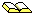Problem 402 in The College Mathematics Journal, vol. 21, No. 4, September 1990, page 339.

For a similar unlikely-looking collection of identities see:Incredible Identities by D Shanks in Fibonacci Quarterly vol 12 (1974) pages 271 and 280.

2, 1, 3, 4, 7, 11, 18, 29, 47, 76, 123, 199, 322, 521, 843 ..More..## The Lucas Numbers in Pascal's Triangle

We found the Fibonacci numbers appearing as sums of "diagonals" in Pascal's Triangle on the Mathematical Patterns in the Fibonacci Numbers page. We can also find the Lucas numbers there too.

Here is the alternative form of Pascal's triangle that we referred to above, with the diagonals re-aligned as columns and the sums of the new columns are the Fibonacci numbers:

 0 1 2 3 4 5 6 7 8 9 0 1 . . . . . . . . . 1 . 1 1 . . . . . . . 2 . . 1 2 1 . . . . . 3 . . . 1 3 3 1 . . . 4 . . . . 1 4 6 4 1 . 5 . . . . . 1 5 10 10 5 6 . . . . . . 1 6 15 20 7 . . . . . . . 1 7 21 8 . . . . . . . . 1 8 9 . . . . . . . . . 1 1 1 2 3 5 8 13 21 34 55
To derive the Lucas numbers we still add the columns, but to each number in the column we first multiply by its column number and divide by its row number! Here's an example:-
Let's take the third column which, when after the appropriate multiplications and divisions should sum to L(3) which is 4. The lowest number in column 3 is 1 and it is on row 3, so we need:
`    1 x column / row = 1 x 3 / 3 = 1 `
which, in this case, doesn't alter the number by much!
The other number in column 3 is 2 on row 2, so this time we have:
`    2 x column / row = 2 x 3 / 2 = 3`
Note that for all the numbers in the same column, we will always multiply by the same number - the column number is the same for all of them - but the divisors will alter each time.
Adding the numbers we have derived for this column we have 1+3=4 which is the third Lucas number L(3).

Here is what happens in column 4, starting from the bottom again:-

```

1 × 4 / 4 = 1
3 × 4 / 3 = 4
1 × 4 / 2 = 2
SUM  = 7

```
Here's our revised Pascal's triangle from above showing some of the fractions that we use to derive the Lucas numbers - it shows the pattern in the multipliers and divisors more easily:
0 1 2 3 4 5 6 7 8 9
0 1
1   1/1=1 2/1=2
2     2/2=1 3/2=3 4/2=2
3       3/3=1 4/3=4 5/3=5 6/3=2
4         4/4=1 5/4=5 6/4=9  4×7/4= 7  1×8/4= 2
5            5/5=1 6/5=6 10×7/5=14 10×8/5=16 ...
6              6/6=1  6×7/6= 7 15×8/6=20 ...
7                1×7/7= 1  7×8/7= 8 ...
8                  1×8/8= 1 ...
1 3 4 7 11 18 29 47 ...

2, 1, 3, 4, 7, 11, 18, 29, 47, 76, 123, 199, 322, 521, 843 ..More..## References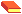Lucas and Primality Testing Hugh C Williams, Wiley, 1998, ISBN: 0471 14852 0
is a new book on how to test if a number is prime without factoring it using a technique developed by Edouard Lucas, with modern extensions to his work.
Primality testing has become a focus of modern number-theory and algorithmics research. Our present inability to find prime factors of a number in a fast and efficient way is relied upon in encryption systems - systems which encode information to send over phone lines. Such encryption systems are now built into computer chips in
• cash-card machines which communicate with your bank's central computing service to check your PIN and to verify the transaction;
• electronic cash transfer over the WWW where your browser encodes the message
• credit card transactions when your card is swiped through a machine at the till
Each of these systems must send the information in a secure way, free from tampering by fraudsters.© 1996-2016 Dr Ron Knott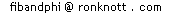updated 23 January 2017